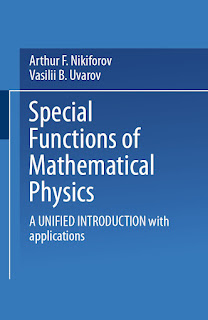APPLIED MATHEMATICS

# Special Functions of Mathematical Physics A Unified Introduction with Applications PDF Free DownloadWith students of Physics chiefly in mind, we have collected the material on special functions that is most important in mathematical physics and quan­tum mechanics. We have not attempted to provide the most extensive collec­tion possible of information about special functions, but have set ourselves the task of finding an exposition which, based on a unified approach, ensures the possibility of applying the theory in other natural sciences, since it pro­ vides a simple and effective method for the independent solution of problems that arise in practice in physics, engineering and mathematics. For the American edition we have been able to improve a number of proofs; in particular, we have given a new proof of the basic theorem (§3). This is the fundamental theorem of the book; it has now been extended to cover difference equations of hypergeometric type (§§12, 13). Several sections have been simplified and contain new material. We believe that this is the first time that the theory of classical or­ thogonal polynomials of a discrete variable on both uniform and nonuniform lattices has been given such a coherent presentation, together with its various applications in physics.